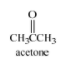# Problem: Consider the structural formula of acetone given below.a) Using Lewis structures for each reactant and each product and using curved arrows appropriately to show the flow of electrons in the reactants, give a chemical equation for the reaction between hydroxide ion (HO− ) and acetone in which HO− acts as a Lewis base and acetone acts as a Lewis acid. IMPORTANT: For this part a of problem 8, the Lewis base that you give cannot also be a Brønsted-Lowry base; the Lewis acid that you give cannot also be a Brønsted-Lowry acid. b) Using Lewis structures for each reactant and each product and using curved arrows appropriately to show the flow of electrons in the reactants, give a chemical equation for the reaction between hydroxide ion (HO− ) and acetone in which HO− acts as a Brønsted-Lowry base dehyde acts as a Brønsted-Lowry acid. Do not give the same answer that you gave in part a of this problem. Recall that for a Lewis structure, you must show all atoms, all bonding valence electrons, all nonbonding valence electrons, and all nonzero formal charges.

###### Problem Details

Consider the structural formula of acetone given below.

a) Using Lewis structures for each reactant and each product and using curved arrows appropriately to show the flow of electrons in the reactants, give a chemical equation for the reaction between hydroxide ion (HO ) and acetone in which HO acts as a Lewis base and acetone acts as a Lewis acid. IMPORTANT: For this part a of problem 8, the Lewis base that you give cannot also be a Brønsted-Lowry base; the Lewis acid that you give cannot also be a Brønsted-Lowry acid.

b) Using Lewis structures for each reactant and each product and using curved arrows appropriately to show the flow of electrons in the reactants, give a chemical equation for the reaction between hydroxide ion (HO ) and acetone in which HO acts as a Brønsted-Lowry base dehyde acts as a Brønsted-Lowry acid. Do not give the same answer that you gave in part a of this problem.

Recall that for a Lewis structure, you must show all atoms, all bonding valence electrons, all nonbonding valence electrons, and all nonzero formal charges.What scientific concept do you need to know in order to solve this problem?

Our tutors have indicated that to solve this problem you will need to apply the Nucleophilic Addition concept. You can view video lessons to learn Nucleophilic Addition. Or if you need more Nucleophilic Addition practice, you can also practice Nucleophilic Addition practice problems.

What is the difficulty of this problem?

Our tutors rated the difficulty ofConsider the structural formula of acetone given below.a) Us...as low difficulty.

How long does this problem take to solve?

Our expert Organic tutor, Jonathan took 8 minutes and 6 seconds to solve this problem. You can follow their steps in the video explanation above.

What professor is this problem relevant for?

Based on our data, we think this problem is relevant for Professor Carberry's class at SJU.